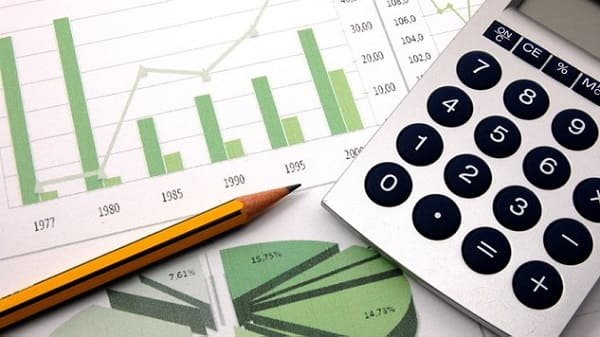# Top 13+ Google Ads PPC Formula with Example for Beginners to ExpertsArticle InShort [INDEX]

The PPC Formula is a quick way to calculate the performance of your campaigns. However, here are the few frequently used PPC Formulas I have shared which are easy to remember and understand the logic. It would definitely be going to help you in calculating your performance of Google Ads with CPC Formula, CPA Formula, CTR Formula, CPM Formula, ROI Formula, ROAS Formula & many more.

So let’s move towards learning the all frequently used PPC Formula of Google Ads Campaigns or PPC Campaigns using Google Ads Formulas: –

### 1. CPC Formula / Cost Per Click Formula

E.g. If I have spent Rs. 1000 & got 500 clicks, then my Cost Per Click Formula / CPC Formula would be;

CPC=1000/500 = Rs.2
Means, My Cost Per Click would be Rs. 2

### 2. CPA Formula / Cost Per Acquisition Formula

E.g. If I have spent Rs. 1000 & got 10 Conversions, then my Cost Per Aquisition Formula would be;

CPA=1000/10 = Rs.100
Means, My Cost Per Acquisition would be Rs.100 or I can say, it costs Rs.100 in a conversion.

### 3. CPM Formula / Cost Per Thousand Impressions Formula

E.g. If I have spent Rs. 1,000 & got 10,000 Impressions, then my CPM Formula would be;

CPM = 1000/ 10000 = Rs.0.1

### 4. CTR Formula / Click Through Rate Formula

E.g. If I have got 500 Clicks & 10,000 Impressions, then my Click Through Rate Formula would be;

=> Click Through Rate = 500/ 10000 *100
=> CTR = 0.05*100
=> CTR = 5%

### 5. CR Formula / Conversion Rate Formula

E.g. If I have got 10 Conversions by getting 100 Clicks, then my CR Formula would be;

=> CR = 10/100 * 100
=> CR = 0.1 * 100
=> CR = 10%

### 6. ROI Formula/ Return on Investment Formula

To clarify, ROI calculated on the basis of your total investment on the product including the manufacturing, transportation, taxes & all other miscellaneous costs. So here, As a spent, the calculation will contain the advertising cost along with the manufacturing and other costs.

E.g. If I have got a revenue of Rs. 10,000 on spending a total of Rs. 1000 on Google Advertising & Rs.4000 on the manufacturing & other investment of my product, then my ROI Formula would be;

ROI = (Total Revenue – (Google Advertising Costs + Product Manufacturing & Other Costs)) / (Google Advertising Costs + Product Manufacturing & Other Costs) * 100

=> ROI = (10000 – (1000+4000)) / (1000+4000) * 100
=> ROI = (10000 – 5000)/ 5000 * 100
=> ROI = 5000/ 5000 * 100
=> ROI = 1*100
=> ROI = 100 %

E.g. If I have got a revenue of Rs. 1,000 on spending a total of Rs. 100 on Google Advertising & Rs.500 on the manufacturing & other investment of my product, then my ROI would be;

ROI = (Total Revenue – (Google Advertising Costs + Product Manufacturing & Other Costs)) / (Google Advertising Costs + Product Manufacturing & Other Costs) * 100

=> ROI = (1000 – (100+500)) / (100+500) * 100
=> ROI = (1000 – 600)/ 600 * 100
=> ROI = 400/ 600 * 100
=> ROI = 0.67*100
=> ROI = 67 %

### 7. ROAS Formula/ Returnon Ad Spend Formula

E.g. If I have got a revenue of Rs. 10,000 on spending a total of Rs. 1,000, then my ROAS Formula would be;

=> ROAS = 10000/ 1000
=> ROAS = 10

That means, in ratio, it is 10:1. So, I can say that my ROAS is 10 times of my spending amounts on running Google Ads Campaigns.

### 8. Quality Score Formula

QS = The combination of Expected Click Through Rate, Keywords Relevancy & Landing Page Experience

E.g. If I am willing to pay a maximum of Rs. 5 for a click & my Quality Score is 10, then my Ad Rank Formula would be;

So, I can say that my Ad Rank is 50.

### 11. Total Cost Formula

Total Cost = Total Clicks * CPC

### 12. Cost Formula (You can also Calculate like this)

Cost = conversion rate * profit margin

### 13. Conversion ratio Formula

Conversion ratio = No of conversions/ No of clicks*100

So these were those popular PPC Formula, which get used by the Google Advertising Agency and other PPC Specialist, who keep optimizing their PPC Campaigns on a regular basis.

Hope you just learned few new things from this blog. Your response will inspire me to share more knowledge with you using my experience and skill. So please like, share & leave your comment below. You can also Contact Us for any query or services such as Google Ads or Content Writing.